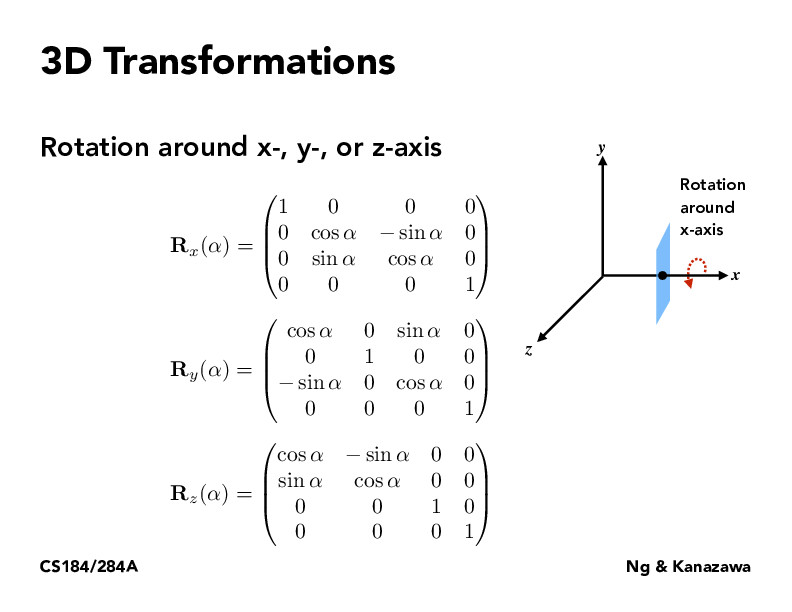Lecture 4: Transforms (46)ochan1

An interpretation of this would be: Pick your rotation around ONE axis

You will affect two of the three axes

Rotation about a 2D space, keep other one constant

In short, you are working to rotate utilizing two axes at a time (mini-proof is notice the columns with the 1s and the 2D rotation like elements in the matrix)yirenng

@ochan1 -- thank you!yirenng

For $\mathbf{R}_y(\alpha)$, where would the red arrow go? And how about for $\mathbf{R}_z(\alpha)$. Why does the top-right $\sin(\alpha)$ in the second matrix lack a negative sign?rollororo

To rotate in an even greater dimension, would we just follow the same patterns of these rotation matrices and also add rotation matrices for the extra dimensions?

You must be enrolled in the course to comment